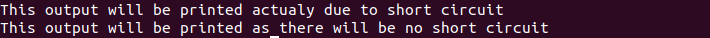# Short-Circuiting in C++ and Linux

Short-circuiting is one of the optimization steps of the compiler, in this step unnecessary calculation is avoided during the evaluation of an expression. Expression is evaluated from left to right. It works under certain cases when the value of the expression can be calculated certainly by only evaluating parts of the expression.

Short-circuiting in C++
In C++ short-circuiting occurs while evaluating ‘&&’ (AND) and ‘||'(OR) logical operators. While evaluating ‘&&’ operator if the left-hand side of ‘&&’ gives false, then the expression will always yield false irrespective of the value of the right-hand side of ‘&&’, so checking right-hand side of ‘&&’ makes no sense. So, in this situation evaluation of the right-hand side is avoided by the compiler. Similarly, in the case of logical OR ‘||’ operation when the left-hand side gives ‘true’, the result of the expression will always be true irrespective of the value of the right-hand side.

Short-circuiting using AND(&&) and OR(||) logical operator.

## C++

 `#include ` `using` `namespace` `std; ` ` `  `int` `main() ` `{ ` `    ``// Short circuiting ` `    ``// logical "||"(OR) ` `    ``int` `a = 1, b = 1, c = 1; ` ` `  `    ``// a and b are equal ` `    ``if` `(a == b || c++) { ` `        ``cout << ``"Value of 'c' will"` `             ``<< ``"  not increment due"` `             ``<< ``" to short-circuit"` `             ``<< endl; ` `    ``} ` `    ``else` `{ ` `        ``cout << ``"Value of 'c' "` `             ``<< ``" is incremented as there"` `             ``<< ``" is no short-circuit"` `             ``<< endl; ` `    ``} ` ` `  `    ``// Short circuiting ` `    ``// logical "&&"(OR) ` ` `  `    ``if` `(a == b && c++) { ` `        ``cout << ``"Value of 'c' will"` `             ``<< ``" increment as there"` `             ``<< ``" is no short circuit"` `             ``<< endl; ` `    ``} ` `    ``else` `{ ` `        ``cout << ``"Value of 'c' will"` `             ``<< ``" not increment due to short circuit"` `             ``<< endl; ` `    ``} ` `} `

Output:

```Value of 'c' will  not increment due to short-circuit
Value of 'c' will increment as there is no short circuit
```

Short Circuiting in Linux

In Linux, short-circuiting takes place while evaluating ‘&&’ and ‘||’ operators just like C++.

Logical AND(&&) short circuiting:

if [[ “\$1” -gt 5 ]] && [[ “\$1” -lt 10 ]]; then
echo “This output will not be printed”
else
echo “This output will be printed”
” actualy due to short circuit”
fi

if [[ “\$1” -lt 5 ]] && [[ “\$1” -lt 10 ]]; then
echo “This output will be printed”
” as there will be no short circuit”
else
echo “This output will be printed”
” actually due to short circuit”
fi

Output:Logical OR(||) short circuiting:

if [[ “\$1” -lt 5 ]] || [[ “\$1” -gt 10 ]]; then
echo “This output will be printed”
” actualy due to short circuit”
else
echo “This output will be printed”
” as there will be no short circuit”
fi

if [[ “\$1” -gt 5 ]] || [[ “\$1” -lt 10 ]]; then
echo “This output will be printed”
” as there will be no short circuit”
else
echo “This output will be printed”
” actually due to short circuit”
fi

Output:Note: ‘&&’ operator is often used as a replacement to ‘if’ statement.

For example, to check for the existence of a file you could use either of the below:

• ```if  [[ -e "\$filename" ]]; then
echo "exists"
fi
```
• ```[[ -e "\$filename" ]] && echo "exists"
```

In this case, bash first executes the expression on the left of the &&(and). If that expression has a non-zero return value (i.e. it failed), then there is no need to evaluate the right side of the && because the overall exit status is already known to be non-zero since both sides have to return a success indicator for a logical and to return success.

Attention reader! Don’t stop learning now. Get hold of all the important DSA concepts with the DSA Self Paced Course at a student-friendly price and become industry ready.

My Personal Notes arrow_drop_upCheck out this Author's contributed articles.

If you like GeeksforGeeks and would like to contribute, you can also write an article using contribute.geeksforgeeks.org or mail your article to contribute@geeksforgeeks.org. See your article appearing on the GeeksforGeeks main page and help other Geeks.

Please Improve this article if you find anything incorrect by clicking on the "Improve Article" button below.

Article Tags :
Practice Tags :

Be the First to upvote.

Please write to us at contribute@geeksforgeeks.org to report any issue with the above content.